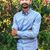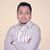# What is the concept of diminishing returns? What role does the Price Line play for the equilibrium condition?

What is the concept of diminishing returns? What role does the Price Line play for the equilibrium condition?

This post was part of TyroCity discussion forumThe law of diminishing returns states that if the unit or quantity of a factor of production is increased continuously, other factors remaining constant, eventually the marginal and average product decline. The law of diminishing returns states that people will get less and less extra output when they add additional doses of an input while holding other inputs fixed (Olesky, 2012). In other words, it states that the marginal and average product increases at the beginning that reaches a maximum point and starts to fall eventually along with a continuous increase in one factor of production(labor), keeping all other factors of production constant.

According to “C.E. Ferguson,” As the amount of variable input is increase, the number of other inputs held constant, a point is reached beyond which marginal product declines".

An assumption of the law of diminishing returns

• The state of technology is given and constant

• The ration in which different factors are combined should be liable to vary

• The quantity of at least one factor should be kept fixed.

• This law is based on short-run production function.

There is three stage of production shown on the table.

First stage: The total product and average product increase rapidly at this stage. The marginal product is maximum when 3 units of labor are used and diminishes thereafter. This stage is called the stage of increasing returns. Because of the more use of variable factor increases its own efficiency and there is enough fixed capital there is an increase in the production in this stage.

Second stage: The total product continues to increase in diminishing rate in this stage. The marginal land average product both decline. This stage ends when the total product is the maximum and marginal product is minimum. This stage is called the stage of diminishing returns. The reason for the decrease in production in this stage is due to the indivisibility of the fixed factor and the fixed factor is over-utilized and becomes inadequate.

Third stage: In this stage, the total product and average product both decline whereas the marginal product is negative. This stage is defined as negative returns because of the unnecessary use of a labor unit alone creates unhealthy competition among the workers. Therefore, a proverb, too many cooks spoils the broth” applies in this case.

This figure shows the law of diminishing returns in which TP, MP, and AP are measured along a ‘Y’- axis. Here the TP curve has increased upward at increasing rate in the first stage and again increased at a decreasing rate in the second stage. It started to fall after a maximum point in stage three along with the increase in the labor unit in production. Similarly, Marginal product (MP) curve increased faster than Average product curve. It continued to increase till stage three and ultimately become negative in stage three along with the increase in labor units in production. Likewise, Average Product (AP) curve increased slower than marginal product and reached maximum point in the first stage. It started to fall in the second stage and continued to fall in the third stage along with an increase in labor units in production.

Thus it is clear from the above table and figure that the law of diminishing returns is the notion that there exists a point where the addition of resources increases production but does so at a decreasing rate (Guell, 2012).

A price line or a budget line is crucial for knowing the theory of consumer’s equilibrium and important element analysis of consumer behavior which represents the various combinations of two goods. The combinations of two goods can be purchased with a given amount of money income and assumed prices of goods.

The budget line can be written algebraically as follows:

PxX + PyY = M

Where Px and Py denote prices of goods X and Y respectively. Similarly, M stands for money income.

In the figure, the line AF shows the various combinations of goods the consumer can purchase which is called the price line or budget line. The slope of the price line indicates how many packets of biscuits a purchaser must give up to buy one more packet of coffee. The given indifference map shows people’s preferences for the combination of two goods. It identifies the options from which the consumer can choose the combination of goods that is the packets of biscuits and packets of coffee.

From the table, it is clear that the higher indifference curve shows a higher level of satisfaction than a lower one. Thus, a consumer always attempts to maximize his satisfaction by trying to reach the highest possible indifference curve. A consumer can by any combination that lies on the budget or price line with his given money and given prices of goods.

References

Guell, R. C. (2012). Firm Production, Cost and Revenue. In R. C. Guell, Issues in Economic Today (Sixth ed., p. 53). New York: McGraw-Hill.

Olesky, J. (2012, Nov). Diminishing Returns, Diminishing Awareness. Professional Safety, 57 (12), 18.According to Brue (1993), "To modern economists, diminishing returns in the most basic sense occurs when marginal product falls as a rising amount of a variable homogeneous input is applied to a fixed input.” As the term "diminish returns” means a reduction on returns, so it applies that at first when the additional input is added then the output also increases at that rate. However, the more and more single input is added then the output tends to increase at decreasing rate (Guell, 2015). It means that at a particular point the output increases but at a slower rate. After that, it starts to diminish the extra output of the group despite the addition of per unit input.

Now, I would like to define the law of diminishing return trough graphical representation.

Figure: Law of Diminishing Return

In the above graph what we can depict is that at point A the production starts as a firm requires workers to yield the output. Then after at point B, additional workers are added to increase the productivity. From point B the outcome slowly starts to increase. Again the input i.e. additional workers are added to increase the productivity which as a result a steeper slope is created. This means that from the point B to A the output has skyrocketed. Since the point C to point D the output has increased but at the decreasing rate despite the addition of workers. This means that from the point D the law of diminishing return prevailed especially holding other factors of production constant.

I would like to illustrate the law of diminishing return trough numerical representation.

Figure: Numerical Illustration of Law of Diminishing Return

In the above table, there consist input (chefs), total output and productivity of extra output of all chefs. As we can see that initially the production commences from zero input and output and as the production takes its course additional input (chefs) i.e. 3 is added which as a consequence yield the output of 10. Likewise, the additional chefs are further added 3 and 5 which as a result yielded 25 and 55 output sequentially. Up to this point (addition of 7 chefs), the production has increased and reached to the optimum point i.e. 55 extra output by all chefs. Furthermore, when the 10 additional input or chefs are added then the production has increased but at a decreasing rate (50 output by the group). Since then each additional chefs or input has increased but at the decreasing rate.

References

Brue, S. L. (1993). Retrospectives: The law of diminishing returns. Journal of Economic Perspectives, 7 (3), 185-192.

Guell, Robert C. (2015). Issues in Economics Today , 7th edition- 2015 ISBN: 978-0078021817